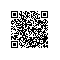# 「BioNano系列」那些Bionano未覆盖的区域是什么？

「Bionano系列」光学图谱混合组装应该怎么做？这篇文章中，我展示了下面这张图。# 利用拟南芥的原本CDS进行注释
gmap_build -D index -d R05C0144 ../R05C0144.fa &
gmap -t 20 -D index -d R05C0144 -f gff3_gene ../Athaliana_cds.fa > cds_gene.gff3 2> log.txt &
# 重复序列注释
RepeatMasker -e ncbi -species arabidopsis -pa 30 -gff -dir . ../R05C0144.fa &
# GFF转成BED
awk 'BEGIN{OFS="\t"} {print $1,$4,$5}' ../repeat_annotation/R05C0144.fa.out.gff > repeat.bed grep -w 'gene' ../gene_annotation/cds_gene.gff3| awk 'BEGIN{OFS="\t"} {print$1,$4,$5}' | bedtools sort -i - > gene.bed
# 统计
bedtools makewindows -w 100000 -g ../R05C0144.txt > windows_100k.bed
bedtools coverage -a windows_100k.bed -b repeat.bed > repeat_stat.bed
bedtools coverage -a windows_100k.bed -b gene.bed > gene_stat.bed

R代码如下

gene_df <- read.table("R05C0144/feature_stat/gene_stat.bed",
sep="\t", stringsAsFactors = F)
sep="\t", stringsAsFactors = F)

options(scipen=999)
contig <- "contig2"

repeat_ctg <- repeat_df[repeat_df$V1 == contig,] gene_ctg <- gene_df[gene_df$V1 == contig,]

combine_df <- data.frame(pos=(repeat_ctg$V2 + repeat_ctg$V3) / 2,
repeat_num=repeat_ctg$V4, gene_num=gene_ctg$V4)
combine_df$total = combine_df$repeat_num + combine_df$gene_num combine_df$gene_ratio <- combine_df$gene_num / combine_df$total * 100

combine_df$repeat_ratio <- combine_df$repeat_num / combine_df$total * 100 plot(combine_df$pos, combine_df$gene_ratio, type="l", ylim=c(0,100), xlab="position", ylab="percent", col="blue") lines(combine_df$pos, combine_df\$repeat_ratio, col="red")
abline(v=7.85*1e6)使用钉钉扫一扫加入圈子
+ 订阅